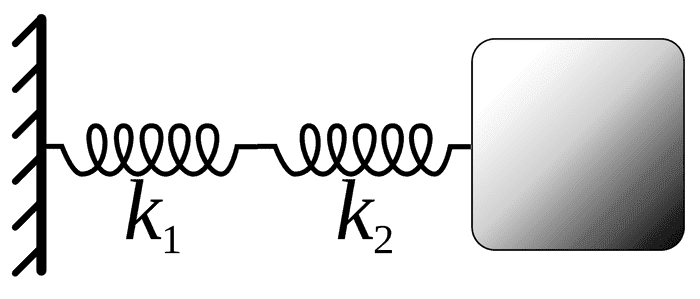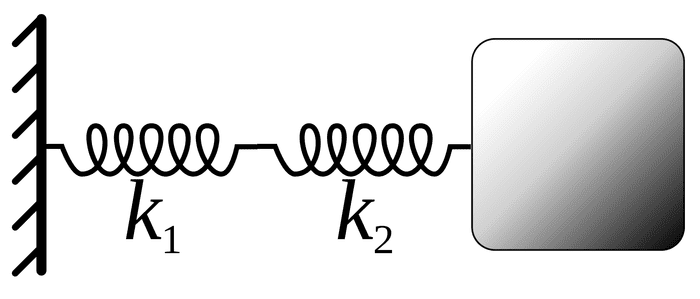# Mass and two springs in series: How to solve acceleration

• Paavo Palikka
In summary, the problem at hand involves two springs in series, spring 1 and spring 2, with spring constants k1 and k2 respectively. The springs are attached to ground and in their initial state, they are not stretched. When a mass is inserted on top of spring 1, the springs start to vibrate. The acceleration between the two springs can be determined using the Lagrangian or Newton's laws, and is given by the second derivative of the displacement of the mass from its equilibrium position. The equivalent spring constant for the series spring system is k1k2/(k1+k2), and the acceleration can be expressed as a function of time, the spring constants, and gravitational force.

#### Paavo Palikka

Hello!

I have rather simple problem, but I can't find somehow answer for it: There are two springs in series, let's say spring 1 and spring 2. Springs are attached and spring 2 is attached to ground. In the beginning springs are not stretched. Let's say springs have spring constants k1 and k2.

When mass is inserted on top of spring 1, springs start to vibrate. What is an acceleration between spring 1 and 2 as a function of time?

Please believe me, this is not a homework but an engineering problem. My skills on basic mechanics are quite rusty :)

I would appreciate if you could show me not only answer but how to solve this, so next time I can solve it myself. Thanks!

-Paavo Palikka

Have you tried to identify the forces acting on the mass? And what do you mean by acceleration between spring 1 and 2?

Well, let's get the Lagrangian for this setup. Let ##x## be the position of the mass and assume that ##x=0## is the equilibrium position. Let ##L_1## and ##L_2## be the springs' length in the equilibrium position. Then we have
$$V=\frac{1}{2} [k_1 (L_1-x)^2+k_2(L_2+x)^2].$$
Then
$$L=T-V=\frac{m}{2} \dot{x}^2 -\frac{1}{2} [k_1 (L_1-x)^2+k_2(L_2+x)^2].$$
The equation of motion is given by the Euler-Lagrange equation
$$\frac{\mathrm{d}}{\mathrm{d} t} \frac{\partial L}{\partial \dot{x}}=m \ddot{x}=\frac{\partial L}{\partial x}=-k_1(x-L_1)-k_2(x+L_2)=-(k_1+k_2) x -(k_2 L_2-k_1 L_1).$$
Since by assumption for ##x=0## the force must vanish, we have ##k_1 L_1=k_2 L_2##, and thus
$$m \ddot{x}=-(k_1+k_2) x.$$
As you see, your setup does not reflect two springs in series but in parallel.

You would have springs in series, if you'd not put the mass between the springs but connecting the strings and fixing the mass on one end of the combined spring and the other end at the ceiling.

vanhees71 said:
You would have springs in series, if you'd not put the mass between the springs ...

How did you infer that the mass is inbetween the springs?Ok the post isn't 100% accurate since it doesn't provide a figure or an attached image of some sort but still from what I can infer the springs are in vertical position in series with spring 1 on top and spring 2 on bottom and mass is on top of the spring system.

Hi again!

my question might be quite unreadable, apologies for that. Unfortunately I can't find any good picture from internet, but maybe picture below is suffisient enough.
One should turn it 90 degrees so that ground is down and springs are vertical. g is acting on mass then vertically.
Acceleration between strings means acceleration between these two strings on picture. Surely it is smaller than acceleration of mass, but how much smaller it is?
I would guess that answer could be given as a function of time t, spring constants k1 and k2 and gravitation g.You mean acceleration of the point between the two springs?
Acceleration between springs does not make much sense. Should be acceleration of some object or even geometric point, if you wish.

Okay, instead of going into Lagrangian let's apply Newton's laws directly but keep in mind that they are equivalent. For a series spring system the equivalent spring constant is k1k2/(k1+k2). You can derive this using the fact that the force on the two springs are equal. If you have difficulty deriving this you can check the wikipedia page posted above. So now we converted the two spring system to one spring.

Now the forces one the mass is clearly a gravitational force and spring force. Let's call the distance of the mass from intial point x. Using Newton's second law mg-k1k2x/(k1+k2)=ma. Since a is the second derivative of x you have a differential equation. After solving this equation for x, you can obtain a by differentiating it twice.

One extra note, you can find the x without finding equivalent k, but this time you need to define x1 and x2 for two springs and find the relationship between x1 and x2(using equal force approach) then the distance of the mass from initial point will be x1+x2. After that you need to write the new equation for the mass since the opposite force will be due to x1 but the acceleration will be second derivative of x1+x2. However you will get the same equation. Hope it helps.

•Delta2
Paavo Palikka said:
Hi again!

my question might be quite unreadable, apologies for that. Unfortunately I can't find any good picture from internet, but maybe picture below is suffisient enough.
One should turn it 90 degrees so that ground is down and springs are vertical. g is acting on mass then vertically.
Acceleration between strings means acceleration between these two strings on picture. Surely it is smaller than acceleration of mass, but how much smaller it is?
I would guess that answer could be given as a function of time t, spring constants k1 and k2 and gravitation g.Then the two springs are in series, and it's simpler to argue with forces. On both springs the same force are acting. Let again ##x## be the displacement of the mass from it's equilibrium position (then you don't need to consider the gravitational force anymore, because it's for ##x=0## exactly compensated by the springs). If ##x_j## is the change of length on spring ##j## (##j \in \{1,2 \}##) then you have
$$x=x_1+x_2$$
and since the forces are the same
$$k_1 x_1=k_2 x_2.$$
The springs together have a constant ##k##, and then you have
$$F=k x = k (x_1+x_2)= k (x_1+k_1 x_1/k_2)=k (1+k_1/k_2) x_1=k \frac{k_1+k_2}{k_2} x_1=k_1 x_1 \; \Rightarrow \; k= \frac{k_1 k_2}{k_1+k_2}.$$

## 1. What is the formula for calculating acceleration in a system of mass and two springs in series?

The formula for calculating acceleration in a system of mass and two springs in series is a = F/m, where F is the net force acting on the mass and m is the mass of the object.

## 2. How do you solve for the net force in this system?

The net force in a system of mass and two springs in series is equal to the sum of the forces acting on the mass. This can be calculated by adding up the individual forces from each spring, which can be found using Hooke's Law (F = kx).

## 3. Can you explain the concept of equilibrium in this system?

In this system, equilibrium refers to the state where the net force acting on the mass is equal to zero. This means that the forces from the two springs cancel each other out, resulting in no acceleration of the mass.

## 4. How does changing the spring constant affect the acceleration in this system?

The spring constant (k) is directly proportional to the acceleration (a) in this system. This means that increasing the spring constant will result in a greater acceleration of the mass, while decreasing the spring constant will result in a lower acceleration.

## 5. What is the relationship between the mass and acceleration in this system?

According to Newton's Second Law of Motion, the acceleration (a) of an object is inversely proportional to its mass (m). This means that as the mass of the object increases, the acceleration decreases, and vice versa.